# Question 6 Which of the following is equal to Inx - 3lny + Inz? In(x –...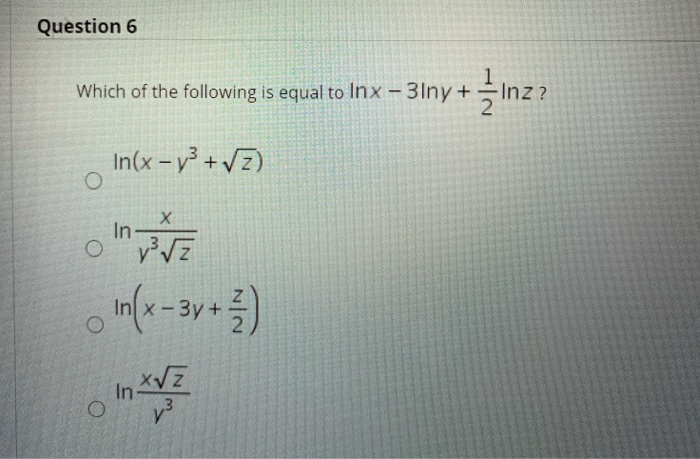Question 6 Which of the following is equal to Inx - 3lny + Inz? In(x – y +V2) in VE In(x - 3y + 4) xyz In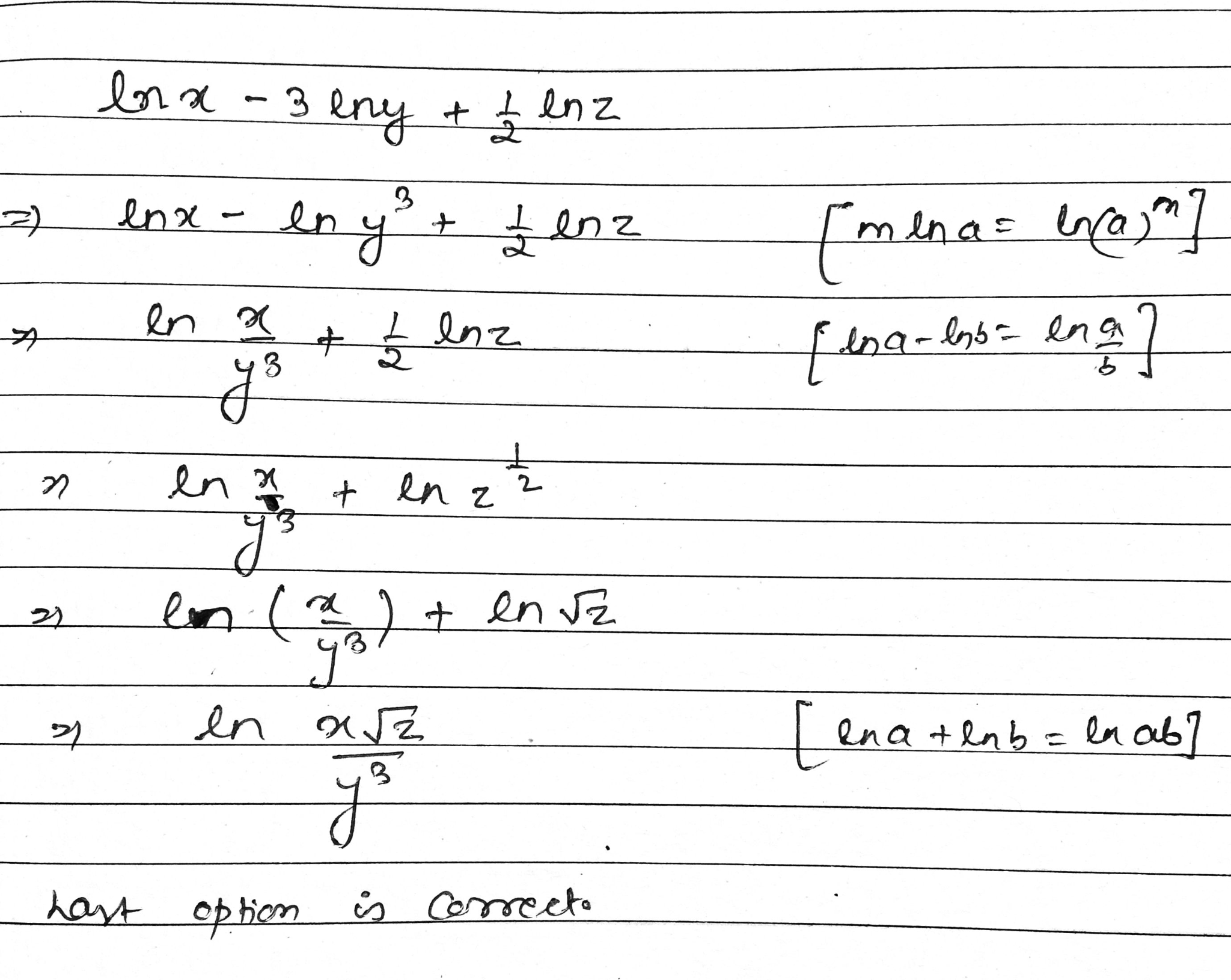##### Add Answer of: Question 6 Which of the following is equal to Inx - 3lny + Inz? In(x –...
Similar Homework Help Questions
• ### Question 4 Given that f(x)= x - 4 (x + 1)(x-3) Which of the following statement...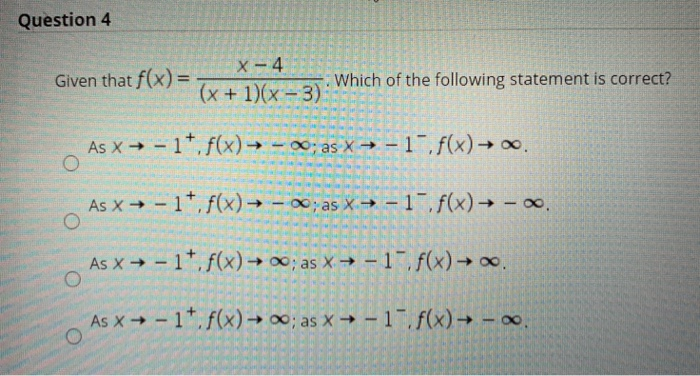Question 4 Given that f(x)= x - 4 (x + 1)(x-3) Which of the following statement is correct? As x → - 17.f(x) — - 20; as X = -1-f(x) → .. As x + - 1*. f(x) — - ~; as x = -1 f(x) - . o As x + - 17. f(x) → 0; as x + -1 f(x) → As → - 17. f(x) → 0; as x + -1 . f(x) - 0. O Question...

• ### 3) Solve the following: max Inx + y x,y ST 2xy 10,x 2 0,y 2 0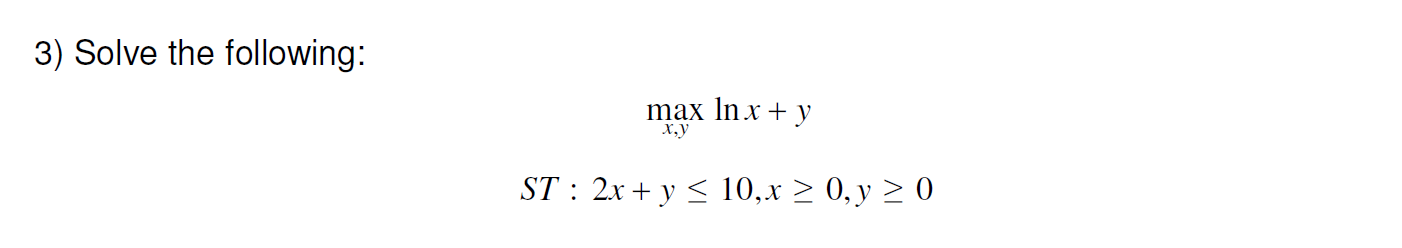3) Solve the following: max Inx + y x,y ST 2xy 10,x 2 0,y 2 0

• ### what would be the anser to this question: find x is greater than or equal to zero and y is greater than or equal to zero such that x + y is less than or equal to 10 and 5x +2y is greater than or equal to 20 and 2y is greater than or equal to x and x + 3y

what would be the anser to this question: find x is greater than or equal to zero and y is greater than or equal to zero such that x + y is less than or equal to 10 and 5x +2y is greater than or equal to 20 and 2y is greater than or equal to x and x + 3y is minimized.Thanks!

• ### Find the derivative of the following functions: Inx 22 C. a. F(x) = In (3x) b....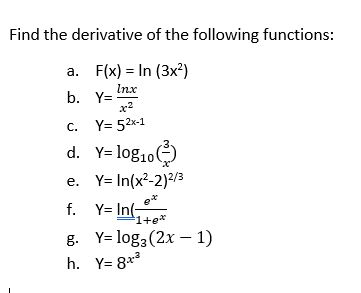Find the derivative of the following functions: Inx 22 C. a. F(x) = In (3x) b. Y=: Y=52x-1 d. Y= log1032 e. Y=In(x2-2)2/3 f. Y= In g. Y= log2 (2x - 1) h. Y= 8** e 1+e+

• ### Question 6 The solution of the differential equation x?y" – 3xy' + 4y = 0) is...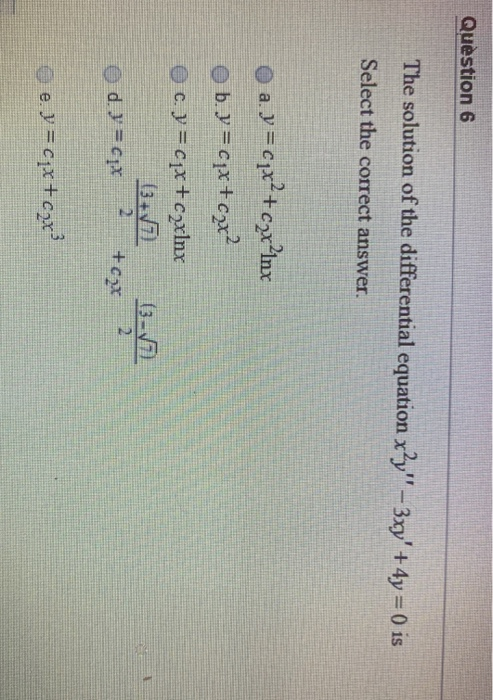Question 6 The solution of the differential equation x?y" – 3xy' + 4y = 0) is Select the correct answer. a. y = cpx?+ czx?inx b. y=cqx+cax? c. y = cqx+c2xlnx (3-5 d = 1x 6-7 +cax e. y = cix+cox?

• ### Solve each system x + 3y = 6 4x - 5y = 7 I need to know what x and y equal

Solve each systemx + 3y = 64x - 5y = 7I need to know what x and y equal??

• ### if absolute value of x-6 is greater than or equal to 4 which of the following must be true

if absolute value of x-6 is greater than or equal to 4 which of the following must be true?A) absolute value of x > 10B) x > 10C) x < 2D) x > -2E) none of the above

• ### how would you solve for y in this problem: x=(y^2+3y)^(1/3) would it equal: y= x^3/y - 3

how would you solve for y in this problem:x=(y^2+3y)^(1/3)would it equal: y= x^3/y - 3 ?and what about this problem:x= 1/[(y^2+3y-5)^3]would it equal: y = y/x + 5/y - 3?I would appreciate your help very much!<<how would you solve for y in this problem:x=(y^2+3y)^(1/3)would it equal: y= x^3/y - 3 ? >>Your equation is correct but it does not allow you to solve explicitly for y. What you have to do is treat the "x-containing" term as if it were...

• ### a/b + b/a = x a/b - b/a = y in the equation above, if a is (not equal) to 0 and b is (not equal) to 0, which of the following must be equal to x^2-y^2

a/b + b/a = xa/b - b/a = yin the equation above, if a is (not equal) to 0 and b is (not equal) to 0, which of the following must be equal to x^2-y^2?a. 2b. 4c. ab/2d. a^2 b^2/2e. 4a^2b^2

• ### a/b + b/a = x a/b - b/a = y in the equation above, if a is (not equal) to 0 and b is (not equal) to 0, which of the following must be equal to x^2-y^2

a/b + b/a = x a/b - b/a = y in the equation above, if a is (not equal) to 0 and b is (not equal) to 0, which of the following must be equal to x^2-y^2? a. 2 b. 4 c. ab/2 d. a^2 b^2/2 e. 4a^2b^2

Free Homework App